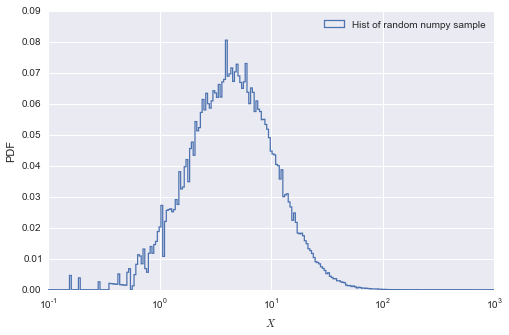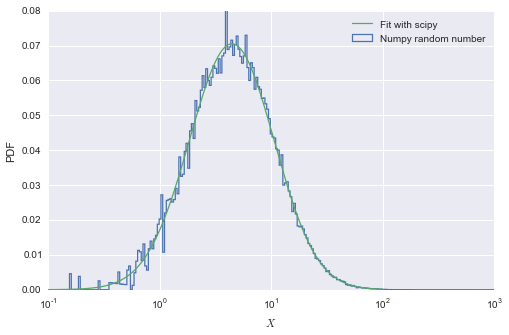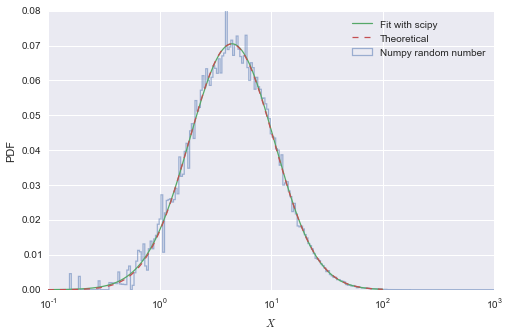Notes:

# TL; DR¶

Using scipy, you can easily use maximum likelihood estimation (MLE) do determine the parameters describing the distribution of a sample of data. However, you can have to get the terminology straight for each type of distribution. This notebook aims to clear that up for lognormal distributions.

If you want to estimate the location $(\mu)$ and scale $(\sigma)$ (as defined by Wikipedia) that describe a lognormally distributed sample $(X)$ with stats.lognorm.fit, do the following:

• fit the distribution, making sure to feed a guess that scipy's loc is 0
• set $\sigma$ to scipy's shape parameter
• set $\mu$ to the natural log of scipy's scale parameter
• check to confirm that scipy's loc parameter is close to 0

If you already know $\mu$ and $\sigma$ of your distribution, then you can create that distribution in the manner below:

myDist = stats.lognorm(sigma, loc=0, scale=numpy.exp(mu))

## More details¶

In :
import numpy
from matplotlib import pyplot
import scipy
from scipy import stats
import seaborn

seaborn.set()
%matplotlib inline

print('numpy: {}'.format(numpy.version.full_version))
print('scipy: {}'.format(scipy.version.full_version))

numpy: 1.9.1
scipy: 0.14.0


## Basic Concepts¶

A (perhaps over-)simplification of the lognormal distirbution would state that a sample $(X)$ is lognormally distributed if $\log(X)$ is normally distributed. This implies that the value of a sample from a populate that is truly distributed lognormally can never be zero. You can get really dang close, but not actually there.

So let's define the parameters that describe the distirbution of $\log(X)$ as $\mu$ (kind of the mean) and $\sigma$ (kind of the standard deviation). In other words:

\begin{equation*} X = e^{(\mu + \sigma \: Z)} \end{equation*}

Where $Z$ is a standard normal variable.

Wikipedia calls these the location and scale. For our purposes, let's change that to wikiLoc and wikiScale. That's a pain in the ass, but it gets worse due to things out of my control, so please bare with me.

## Sample statistics¶

In addition to $\mu$ and $\sigma$, we can also talk about the sample mean and the sample standard deviation. Let's call them wikiM and wikiS.

In python you can compute those very easily with e.g., numpy.mean(X)

## Generate a random sample from numpy¶

You can generate a random sample from a lognormal distribution using numpy and wikiLoc and wikiScale. The wording of the arguments (mean and sigma) is unfortunate, but I don't expect that to change anytime soon.

In :
numpy.random.seed(0)
N = 37000
wikiLoc = 2.25
wikiScale = 0.875

# _n denotes numpy
X_n = numpy.random.lognormal(mean=wikiLoc, sigma=wikiScale, size=N)


### Plot the histogram¶

In :
bins = numpy.logspace(-1, 3, num=250)

fig, ax = pyplot.subplots(figsize=(8, 5))
_ = ax.hist(X_n, bins=bins, cumulative=False, histtype='step',
normed=True, lw=1.25, label='Hist of random numpy sample')

ax.set_xscale('log')
ax.set_xlabel(r'$X$')
ax.set_ylabel('PDF')
ax.legend()

Out:
<matplotlib.legend.Legend at 0x91d6e80>## Enter scipy the confusion¶

We can fit distributions to samples using scipy. The problem is that the terminology is pretty ambiguous. Scipy needs three parameters to fully define a lognormal distribution. Here they are with sp as a prefix to differentiate them from Wikipedia's parameters:

• spShape
• spLoc
• spScale.

You might be thinking: spShape is weird, but the rest make sense.

## They don't make sense.¶

• spShape actually corresponds to wikiScale.
• Remember when we said that lognormal samples can't take values at or below 0? Scipy disagrees. spLoc is used to translate the PDF of $X$ anywhere along the x-axis. In other words, spLoc (almost) always should be set to 0
• spScale doesn't directly coorespond to wikiLoc. It's actually $\log(\mathtt{wikiLoc})$

The take away here is that if you have real-world sample that you think may be best described by a lognormal distribution, don't just throw it into scipy.stats.lognorm.fit and you can't just take the returned parameters at face value.

## Fitting lognormal distributions with scipy¶

When using the .fit method of scipy.stats distributions, you can guide the parameters by specifying a start/guess value. For us, this means doing:

stats.lognorm.fit(mydata, loc=0)

In :
spShape, spLoc, spScale = stats.lognorm.fit(X_n, loc=0)
ln_dist = stats.lognorm(spShape, spLoc, spScale)
print("""
spShape = {:.3f}
spLoc = {:.3f}
spScale = {:.3f}
""".format(spShape, spLoc, spScale))

spShape = 0.866
spLoc = -0.044
spScale = 9.505



So as we expected, this doesn't really makes sense at facevalue. But information we can use is in there somewhere.

### Another graph, this one with the theoretical distribution we just fit¶

In :
fig, ax = pyplot.subplots(figsize=(8, 5))
_ = ax.hist(X_n, bins=bins, cumulative=False, histtype='step',
normed=True, lw=1.25, label='Numpy random number')

# theoretical PDF
x_hat = numpy.logspace(-1, 2, num=1000)
y_hat = ln_dist.pdf(x_hat)

ax.plot(x_hat, y_hat, '-', lw=1.25, label='Fit with scipy')
ax.set_xscale('log')
ax.set_xlabel(r'$X$')
ax.set_ylabel('PDF')
ax.legend()

Out:
<matplotlib.legend.Legend at 0xa766358>See? Buried in all that nonsense is really good info. Let's get it.

## Estimating sample statistics¶

So let's estimate our sample statistics both directly and from the fit theoretical formulas in the wiki article.

\begin{equation*} m = e^{\mu + \frac{1}{2}\sigma^2} \end{equation*}\begin{equation*} s = \sqrt{\left(e^{\sigma^2} - 1 \right) e^{2\mu + \sigma^2}} \end{equation*}
In :
wikiM = numpy.exp(wikiLoc + 0.5*wikiScale**2)
wikiM_est = X_n.mean()

wikiS = numpy.sqrt((numpy.exp(wikiScale**2) - 1)  * numpy.exp(2*wikiLoc + wikiScale**2))
wikiS_est = X_n.std()

summary = """
\tTheory\tSample
wikiM\t{0:.3f}\t{1:.3f}
wikiS\t{2:.3f}\t{3:.3f}
"""
print(summary.format(wikiM, wikiM_est, wikiS, wikiS_est))

	Theory	Sample
wikiM	13.913	13.804
wikiS	14.922	14.748



Everything is pretty close. Cool.

## Now let's compute wikiLoc and wikiScale from our sample estimates¶

In :
sampleLoc = numpy.log((wikiM_est**2) / numpy.sqrt(wikiS_est**2 + wikiM_est**2))
sampleScale = numpy.sqrt(numpy.log(1 + (wikiS_est/wikiM_est)**2))

summary = """
\tTheory\tSample
wikiLoc  \t{0:.3f}\t{1:.3f}
wikiScale\t{2:.3f}\t{3:.3f}
"""

print(summary.format(wikiLoc, sampleLoc, wikiScale, sampleScale))

         	Theory	Sample
wikiLoc  	2.250	2.244
wikiScale	0.875	0.873



Again. Pretty close so we're cool.

## The crux - making sense of the results of scipy's .fit method¶

Recall what scipy returned:

In :
print("""
spShape = {:.3f}
spLoc = {:.3f}
spScale = {:.3f}
""".format(spShape, spLoc, spScale))

spShape = 0.866
spLoc = -0.044
spScale = 9.505



But we want the estimates of wikiLoc (known = 2.25) and wikiScale (0.875) from the scipy fit.

In :
sp_wikiLoc = numpy.log(spScale)
sp_wikiScale = spShape

summary = """
\tTheory\tSample\tScipy
wikiLoc  \t{0:.3f}\t{1:.3f}\t{2:.3f}
wikiScale\t{3:.3f}\t{4:.3f}\t{5:.3f}
"""
print(summary.format(wikiLoc, sampleLoc, sp_wikiLoc, wikiScale, sampleScale, sp_wikiScale))

         	Theory	Sample	Scipy
wikiLoc  	2.250	2.244	2.252
wikiScale	0.875	0.873	0.866



Phew. It all makes sense.

## Now work backwards¶

So now we can use our original wikiLoc and wikiScale values to freeze a stats.lognorm object.

In :
frozen_dist = stats.lognorm(wikiScale, loc=0, scale=numpy.exp(wikiLoc))


### And then plot it¶

But the theoretical and fit distributions are so close we can't really see the difference.

In :
fig, ax = pyplot.subplots(figsize=(8, 5))
_ = ax.hist(X_n, bins=bins, cumulative=False, histtype='step',
normed=True, lw=1.25, alpha=0.5,
label='Numpy random number')

# theoretical PDF
x_hat = numpy.logspace(-1, 2, num=1000)
y_hat_1 = ln_dist.pdf(x_hat)
y_hat_2 = frozen_dist.pdf(x_hat)

ax.plot(x_hat, y_hat_1, '-', lw=1.25, label='Fit with scipy')
ax.plot(x_hat, y_hat_2, '--', lw=1.25, label='Theoretical')
ax.set_xscale('log')
ax.set_xlabel(r'$X$')
ax.set_ylabel('PDF')
ax.legend()

Out:
<matplotlib.legend.Legend at 0xa71b4e0>